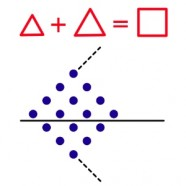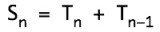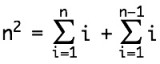# Iconic AlgebraThe Image shows a relationship between whole numbers.  The array of dots is an iconic proof by observation that two successive Triangle Numbers add up to a Square Number, for example, 6+10=16.  In symbolic notation:Symbolic notation becomes cryptic to a non-mathematician when it is written in a form that facilitates symbolic proof:## Algebra and Arithmetic

An arithmetic describes the objects and operations of a mathematical system in terms of the specific objects themselves. The whole number system, for example, has numbers as objects and addition and multiplication as operations on numbers. But the only way to describe how the system works in arithmetic is to list out all the relations between numbers, concrete statements like 1*3=3. When variables are added to an iconic arithmetic, it becomes an iconic algebra. In moving to an algebra the key concept is a variable that stands in place of any arbitrary object in the arithmetic. Variables permit us to say that 1 times anything is the same as that anything, 1*A=A.  Mixing symbolic variables with iconic structure may seem like a hybrid notation, but the concept of an arbitrary form, whether it be symbolic or iconic, is too valuable to be limited to symbolic systems alone.  Iconic variables stand in place of arbitrary iconic forms.  Since the operations of iconic arithmetic are physical actions rather than symbolic rearrangements, iconic variables range over both the intentions and the results of mathematical activity.

## Types of Iconic Algebra of Numbers

The Principles of Iconic Arithmetic can be stated in general for all iconic forms using algebraic variables, generating the Rules of Iconic Algebra.  In this section, several pages show different types of iconic algebras, all sharing the same fundamental principles. The principles are organized into different collections of axioms, each describing how a particular system works.

Container Algebra presents the theory of iconic numbers expressed as equations that can be interpreted as physical instructions to act or as abstract algebraic structures.

Spatial Algebra provides a different type of iconic algebra expressed as physical blocks.  In spatial algebra addition is putting blocks into the same space, while multiplication is represented by putting blocks in contact.

James Algebra is an entirely different boundary-based algebra that incorporates three types of containers to represent real numbers as well as whole numbers.  James containers express exponential and logarithmic concepts in a very convenient manner.  Addition is putting into the same additive space, while multiplication is putting into the same logarithmic space.

The James Imaginary is a new type of imaginary number, the logarithm of –1. The common imaginary, i, is composed of two James imaginaries. The James imaginary leads to new and relatively unexplored ideas such as the generalized inverse, a single operator that achieves all of the common inverse operations (subtraction, division, root).

### Visual Mathematics

One of the early motivations for developing iconic math came from the visual languages community in Computer Science. There is particular interest in developing visual programming languages that may make the task of programming easier. Jeff James and I worked on this problem and published the article A Boundary Notation for Visual Mathematics in the Proceedings of IEEE Visual Langauges’92. Here is the abstract:

Instead of traditional mathematical notation, we can describe formal mathematical systems in visual form. While traditional notation uses a linear sequence of symbols, visual mathematics uses boundary notation, which is comprised of objects and boundaries to enclose objects. Boundary notation is abstract, decoupling the underlying mathematics of a system from its visual representation. Once a system is defined in boundary notation, visual designs can be explored that optimize specific features. We demonstrate this approach with propositional logic and elementary algebra. Visual mathematics provides a robust foundation for visual languages much as linear mathematics provides a foundation for programming languages.